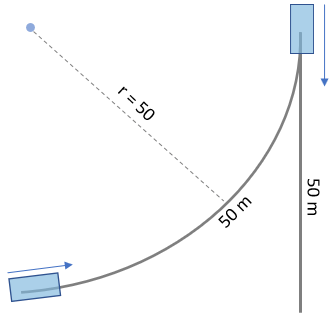Velocity Profile of Path with Curve and Direction Change

This model uses a Velocity Profiler block to generate a velocity profile for a driving path that includes a curve and a change in direction. In this model, the vehicle travels forward on a curved path for 50 meters, and then travels straight in reverse for another 50 meters.The Velocity Profiler block generates velocity profiles based on the speed, acceleration, and jerk constraints that you specify using parameters. You can use the generated velocity profile as the input reference velocities of a vehicle controller.

This model is for illustrative purposes and does not show how to use the Velocity Profiler block in a complete automated driving model. To see how to use this block in such a model, see the Automated Parking Valet in Simulink example.

Open and Inspect Model

The model consists of a single Velocity Profiler block with constant inputs. Open the model.

model = 'VelocityProfileCurvedPathDirectionChanges';
open_system(model)The first three inputs specify information about the driving path.

• The Directions input specifies the driving direction of the vehicle along the path, where 1 means forward and –1 means reverse. In the first path segment, because the vehicle travels only forward, the direction is 1 along the entire segment. In the second path segment, because the vehicle travels only in reverse, the direction is –1 along the entire segment.

• The CumLengths input specifies the length of the path. The path consists of two 50-meter segments. The first segment represents a forward left turn, and the second segment represents a straight path in reverse. The path is composed of a sequence of 200 cumulative path lengths, with 100 lengths per 50-meter segment.

• The Curvatures input specifies the curvature along this path. The curvature of the first path segment corresponds to a turning radius of 50 meters. Because the second path segment is straight, the curvature is 0 along the entire segment.

In a complete automated driving model, you can obtain these input values from the output of a Path Smoother Spline block, which smooths a path based on a set of poses.

The StartVelocity and EndVelocity inputs specify the velocity of the vehicle at the start and end of the path, respectively. The vehicle starts the path traveling at a velocity of 1 meter per second and reaches the end of the path traveling at a velocity of –1 meters per second. The negative velocity indicates that the vehicle is traveling in reverse at the end of the path.

Generate Velocity Profile

Simulate the model to generate the velocity profile.

out = sim(model);

The output velocity profile is a sequence of velocities along the path that meet the speed, acceleration, and jerk constraints specified in the parameters of the Velocity Profiler block.

The block also outputs the times at which the vehicle arrives at each point along the path. You can use this output to visualize the velocities over time.

Visualize Velocity Profile

Use the simulation output to plot the velocity profile.

t = length(out.tout);
velocities = out.yout.signals(1).values(:,:,t);
times = out.yout.signals(2).values(:,:,t);

plot(times,velocities)
title('Velocity Profile')
xlabel('Time (s)')
ylabel('Velocity (m/s)')
annotation('textarrow',[0.63 0.53],[0.56 0.56],'String',{'Direction change'});
grid onFor this path, the Velocity Profiler block generates two separate velocity profiles: one for the forward left turn and one for the straight reverse motion. In the final output, the block concatenates these velocities into a single velocity profile.

A vehicle that follows this velocity profile:

1. Starts at a velocity of 1 meter per second

2. Accelerates forward

3. Decelerates until its velocity reaches 0, so that the vehicle can switch driving directions

4. Accelerates in reverse

5. Decelerates until it reaches its ending velocity

In both driving directions, the vehicle fails to reach the maximum speed specified by the Maximum allowable speed (m/s) parameter of the Velocity Profiler block, because the path is too short.

For details on how the block calculates the velocity profile, see the Algorithms section of the Velocity Profiler block reference page.